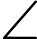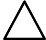Mathematics 1 And Physical Science October/November 2015

Exam Year:2015

This past paper applies to

INSTRUCTIONS TO CANDIDATES
Write your name and index number in the space provided above.
Sign and write the date of examination in the space provided above.
You should have Mathematical table/Scientific calculator and drawing instruments for this examination
This paper consists of EIGHT questions in TWO sections: A and B.
Answer FIVE questions, choosing TWO questions from section A, TWO questions from section B and ONE question from either section in the space provided in this question paper.
All questions carry equal marks.
Maximum marks for each part of a question are as shown.
Do NOT remove any pages from this question paper.
Candidates should answer the questions in English.

SECTION A:MATHEMATICS
Answer at least TWO questions from this section.
(a) (i) Transpose the formulaTo make r the subject.
(3 marks)

(ii) Solve the simultaneous equations
8x -3y = -39
7x+ 6y =9
(3 marks)
(iii) Simplify(3 marks)

(b) Solve the equation(3 marks)
(c) Find the sum of all the numbers between 6 and 204 which are exactly divisible by 3.
(5 marks)

(a) Draw a triangle ABC such that AB =10 cm, AC= 4cm,BAC=90o. Construct a triangle ABP such thatBAP=60o andAPB =90o. Measure AP and PB.
Calculate area ofABC.
(10 marks)

(b) solve the equationFor 0o=0 = 360
(8 marks)
(c) A pyramid with a square base has an altitude of 15 cm. If the side of the square is 6 cm,find its volume.
(2 marks)

(a) Draw a triangle ABC with AB=10cm, AC =4cm andBAC= 90o. Construct a triangle ABP such thatBAP = 60o andAPB = 90o. Measure PA and PB.
Calculate the area ofABC.
(10 marks)
(b) P and Q are two points on latitude 62oN. They lie on longitude 40oW and 140oE respectively. Determine the distance P to Q:
Along a parallel of latitude;
A long a great circle.
Take the radius of the earth to be 6400 km.
(10 marks)

(a) The probability of the occurrence of three events x,y and z are 2544 and 58 respectively. Determine the probability of:
All events occurring;
Event x and y but not z occurring;
Only event y occurring;
None of the events occurs.
(8 marks)

(b) Table 1 gives the distribution of monthly income of 100 workers in a certain construction firm:
TABLE 1
Match the following.

 Monthly Income No. of workers (Ksh. ’00)) 0-75 8 75-150 10 150-225 20 225-300 22 300-375 18 375-450 12 450-525 10

Determine the:
(i) Mean;
(ii) Median;
(iii) Standard deviation.
(12 marks)

SECTION B: PHYSICAL SCIENCE
Answer at least TWO questions from this section.
(a) Name the following covalent compounds:
(i) P2O5;
(ii) Cl2O7;
(iii) Cl2.
(3 marks)
(b) Explain two uses of graphite in relation to its physical properties.
(6 marks)
(c) Describe using a chemical equation, how chlorine can be used to convert iron (II) ions (Fe2+) into iron (III) irons ( Fe3+).
(8 marks)
(d) Given three examples of common reducing agents.
(3 marks)
(a) Give the names of six different types of naturally occurring polymers.
(6 marks)
(b) Name TWO natural and two synthetic fibres.
(4 marks)
(c) Describe three applications of isotopes.
(6 marks)

(d) A trumpet is placed at a distance of 3.2 m from a wall. Assuming that the sound waves produced by the trumpet are spherical,determine the intensity of the sound waves produced by the trumpet, when the power of the trumpet is 0.20 W.
(4 marks)
(a) A convex mirror of focal length 18 cm produces an image on its axis 12 cm away from the mirror. If the image is 6 cm high, by scale drawing,determine the
Object distance from the mirror;
Size of the object.
(9 marks)
(b) A light steel bar 8 m long is supported at both ends by sharp – edged supports.The bar is subjected to vertical downwards forces of 32N and 24N at 2 m and 4 m from the left–hand support respectively. Calculate the reactions at the sharp- edge supports.
(11 marks)

(a) Describe three characteristics of an acid.
(6 marks)
(b) Briefly state the difference between the following:
Deliquescent and efflorescent salt;
Hydrated salts and anhydrous salts
(8 marks)
(c) Describe three properties of covalent compounds.
(6 marks)